• 01背包问题贪心算法
2021-05-24 03:54:35

for iß1 to n do ／／将所有结点标记为未访问／／

repeat

for i

if visited(i)＝0 then ⑵ endif

repeat

end bft

2．找一个图的所有m—着色方案

procedure mcoloring(k)

／／这是图着色的一个递归回溯算法。图g用它的布尔邻接矩阵graPh(1：n，1：n)表示。它计算并打印出符合以下要求的全部解，把整数1，2，…，m分配给图中各个结点且使相邻近的结点的有不同的整数。k是下一个要着色结点的下标。／／

global integer m，n，x(1：n)boolean graPh(1；n，1：n)

integer k

loop／／产生对x(k)所有的合法赋值。／／

call nextvalue(k)。//将一种合法的颜色分配给x(k)／／

if ⑴ then exit endif ／／没有可用的颜色了／／

if ⑵

then print(x) ／／至多用了m种颜色分配给n个结点／／

else callmcoloring

endif

repeat

end mcoloring

三、问答题

1.二分查找的思想是什么？

2．请用递归方法写出归并排序法的主要思想和算法。

3.已知如下多段图，请用动态规划方法的向后处理法写出求解此问题的递推公式并完成对各结点的计算。

4. 最小自然数：求具有下列两个性质的最小自然数n：

(１)n的个位数是６；

(２)若将n的个位数移到其余各位数字之前，所得的新数是n的４倍。

提示：仍用穷举法寻找，当找到一个符合条件者便停止。“找到便停止”的重复，宜采用repeat－until循环。

5. 以二叉链表为存储结构，分别写出求二叉树结点总数及叶子总数的算法。

本文来自电脑杂谈，转载请注明本文网址：

http://www.pc-fly.com/a/tongxinshuyu/article-23877-13.html

更多相关内容
• 01背包问题贪心算法，详细解析，令你很快懂的01背包问题中的贪心算法思想
• 贪心问题中有很多典型的例子，此次背包问题，助大家理解该算法
• 贪心算法编写的01背包问题 用c语言编写 附上实验结果及代码
• 主要介绍了JS基于贪心算法解决背包问题,简单说明了贪心算法的概念、原理,并结合具体实例形式分析了JS使用贪心算法解决部分背包问题的具体操作技巧,需要的朋友可以参考下
• 贪心算法求解背包问题 #include<stdio.h> #define maxnumber 20 typedef struct node { float w; float v; int i; }Object; float find(Object wp[],int n,float M) { float x[maxnumber]; int i; float maxprice=0;...
• 贪心算法解决背包问题，用netbeans做的。百分百下载就可以直接运行。JAVA 源文件。
• Python编写的，利用贪心算法解决活动安排、哈夫曼编码、背包问题、最电路径、最优装载、最小生成树等问题
• 物品课分割的装载问题称为背包问题，物品不可分割的装载问题称为0-1背包问题。 #include #include using namespace std; //按性价比贪心策略 typedef struct three { double w; //重量 double v; //价值 double p...
• 问题描述： 假设山洞中有n种宝物，每种宝物...0-1背包问题详见01背包问题_Player_HA的博客-CSDN博客 数据结构及初始化，将n种宝物的重量和价值存储在结构体three(包含重量、价值、性价比3个成员)中，同时求出每种宝物

问题描述：

假设山洞中有n种宝物，每种宝物有一定重量w和相应的价值v，毛驴运载能力有限，只能运走m重量的宝物，一种宝物只能拿一样，宝物可以分割。那么怎么才能使毛驴运走宝物的价值最大呢？

问题分析：

与0-1背包问题类似，所不同的是在选择物品装入背包时，物品可分割，因此可按性价比（价值/质量）排序选择不同的物品。

0-1背包问题详见01背包问题_Player_HA的博客-CSDN博客

数据结构及初始化，将n种宝物的重量和价值存储在结构体three(包含重量、价值、性价比3个成员)中，同时求出每种宝物的性价比也存储在结构体three中，将其按照性价比从高到低排序。采用sum来存储毛驴能够运走的最大价值，初始化为0。

例如：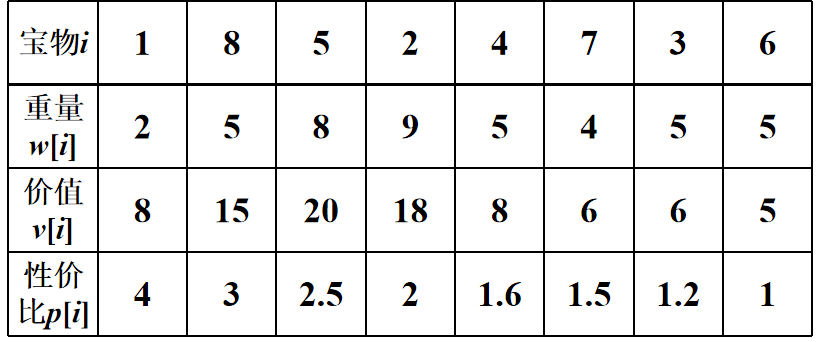代码：

#include<iostream>
#include<algorithm>
using namespace std;
const int M = 100000;
//背包结构体
struct three {
double w;//weight
double v;//value
double p;//性价比
}S[M];

bool cmp(three a, three b)//添加比较规则
{
return a.p > b.p;
}//降序排性价比

int main()
{
int n;//n个物品
double m;//承载能力
cout << "输入物品数量和承载能力：" << endl;
cin >> n >> m;
cout << "输入每个宝物的重量和价值：" << endl;
for (int i = 0; i < n; i++) {
cin >> S[i].w >> S[i].v;
S[i].p = S[i].v / S[i].w;//性价比
}
sort(S, S + n, cmp);//调用库中的函数sort cmp为比较方法
double sum = 0.0;//表示贪心记录运走宝物的价值
int m1 = m;
for (int i = 0; i < n; i++) {
if (m > S[i].w) {
m -= S[i].w;
sum += S[i].v;
}
else {
sum += S[i].p * m;
break;//容器已经装满，跳出循环
}
}
cout << "装得的最大价值：" << sum << endl;
cout << "装得的物品性价比："  << endl;
for (int i = 1; i <=n; i++)
{
cout << "["<<i<<"]:" << S[i].p << endl;
}

}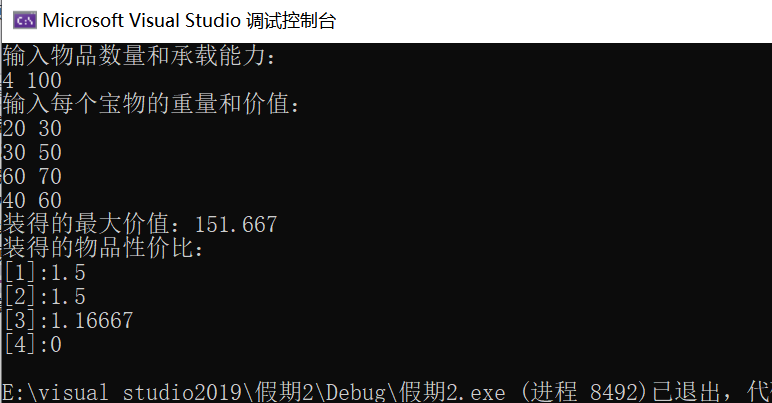展开全文数据结构 c语言 c++
• 贪心算法的基本思想 •贪心算法的特点是每个阶段所作的选择都是局部最优的，它期望通过所作的局部最优选择产生出一个全局最优解。 贪心与动态规划：与动态规划不同的是，贪心是鼠目寸光；动态规划是统揽全局。 ...

## 此算法用冒泡排序和选择排序实现的！！

### 贪心算法的基本思想

•贪心算法的特点是每个阶段所作的选择都是局部最优的，它期望通过所作的局部最优选择产生出一个全局最优解。

贪心与动态规划：与动态规划不同的是，贪心是鼠目寸光动态规划是统揽全局

贪心：每个阶段产生的都是局部最优解

### 贪心算法的基本要素

•贪心选择性质：所求问题的全局最优解可以通过一系列局部最优的选择（即贪心选择）来达到。

–这是贪心算法与动态规划算法的主要区别。

•最优子结构性质：当原问题的最优解包含子问题的最优解时，称此问题具有最优子结构性质。

最优子结构性质是该问题可用动态规划算法或贪心算法求解的关键特征

## 贪心算法：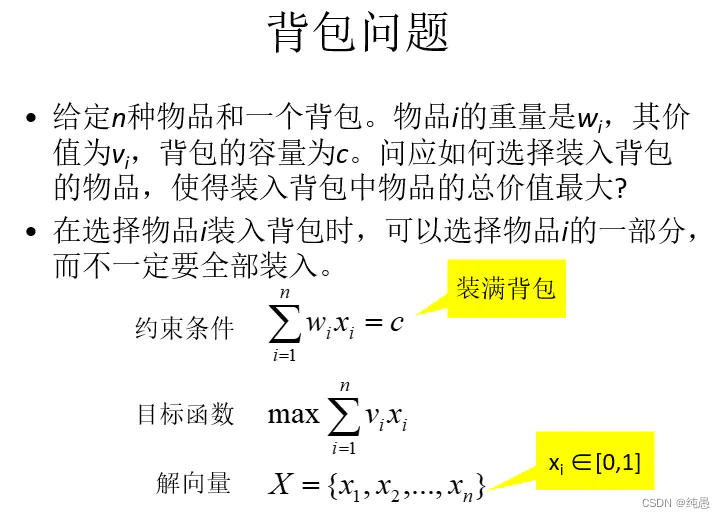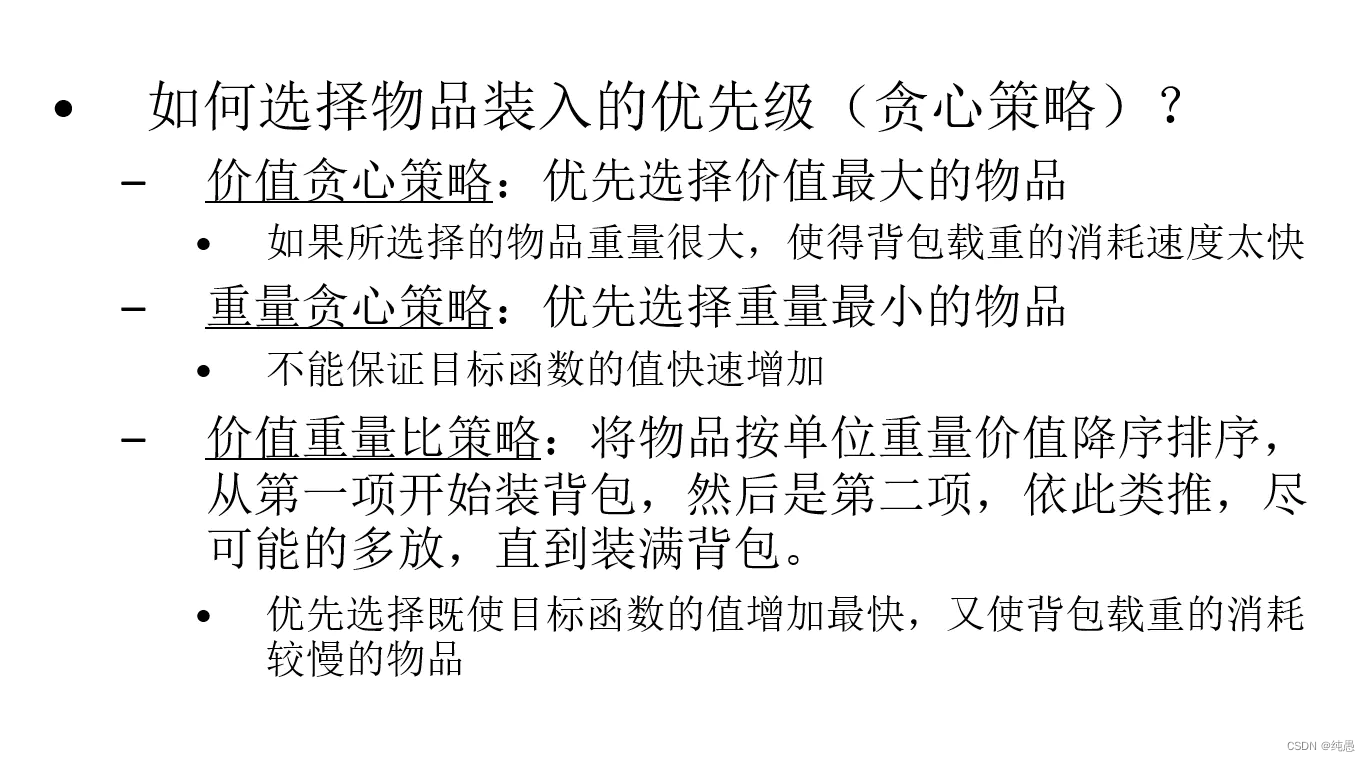### 完整代码：

运行：

3 50

10 20 30

60 100 120

3 50

30 10 20

120 60 100

#include<bits/stdc++.h>
using namespace std;
//冒泡排序
//void Sort(int n,double w[],double v[])
//{
//    int i,j;
//    float temp1,temp2;
//    for(i=1;i<=n;i++){
//    	for(j=1;j<=n-i;j++)//冒泡排序
//    	{
//    	    temp1=v[j]/w[j];
//    	    temp2=v[j+1]/w[j+1];
//    	    if(temp1<temp2)
//    	    {
//    	        swap(w[j],w[j+1]);
//    	        swap(v[j],v[j+1]);
//    	    }
//    	}
//	}
//}

//插入排序
void Sort1(int n,double w[],double v[]){
int max1;
double t;
for(int i=1;i<=n;i++){
t[i]=v[i]/w[i];
//cout<<t[i]<<endl;
}
for(int i=1;i<=n;i++){
max1=i;
for(int j=i+1;j<=n;j++){
if(t[j]>t[max1]){
max1=j;
}
}
swap(t[i],t[max1]);
swap(w[i],w[max1]);
swap(v[i],v[max1]);
}
for(int i=1;i<=n;i++){
cout<<t[i]<<endl;
}
}
//n为物品数量，c为背包最大容量，w[]为物品重量，v[]为物品价值，b[]为背包存放
void knapsack_greedy(int n,double c,double w[],double v[],double b[]){
int j;
//Sort1(n,w,v);
Sort1(n,w,v);
for(int i=1;i<=n;i++)//初始化背包b[]
b[i]=0;
for(j=1;j<=n;j++){
if(c<w[j]) break;
b[j]=1;
c=c-w[j];
}
if(j<=n)
b[j]=c/w[j];
cout<<"输出："<<endl;
for(int i=1;i<=n;i++){
cout<<"物品重量为："<<w[i]<<" "<<"物品比例"<<b[i]<<endl;
}
}
int main(){
int n,c;
double b,w,v;
cout<<"输入物品数量和背包容量：";
cin>>n>>c;
cout<<"输入物品重量（如：30 10 20）：";
for(int i=1;i<=n;i++)
cin>>w[i];
cout<<"输入物品的价值（如：120 60 100）：";
for(int i=1;i<=n;i++)
cin>>v[i];
knapsack_greedy(n,c,w,v,b);
return 0;
}

代码解析：

//Sort1(n,w,v);
Sort1(n,w,v);

1.此函数用来在计算单位价值后排序，使得按照最大单位价值来从大到小排序（然后依次装入背包）

for(int i=0;i<n;i++)//初始化背包b[]
b[i]=0;
for(j=0;j<n;j++){
if(c<w[j]) break;
b[j]=1;
c=c-w[j];
} 

2.首先这是0/1背包问题，在数组b[]中存放的就是0或者1。第一个循环语句初始化为0，表示还没有装物品。

0或1表示的是：物品一整个全部装进去物品比例为1，完全没有装进去物品比例为0，如果背包容量未满，还能装下一个物品的一部分，那么就用剩余的背包容量/这个物品的重量（装进去的物品比例 ：c/w[i] )---为小数（标红句子看下述代码）。

if(j<=n)
b[j]=c/w[j];

在退出循环后，继续判断，物品还未装完，用剩余的背包容量/这个物品的重量（c/w[i])---为小数，也可能为0。

### 代码结果：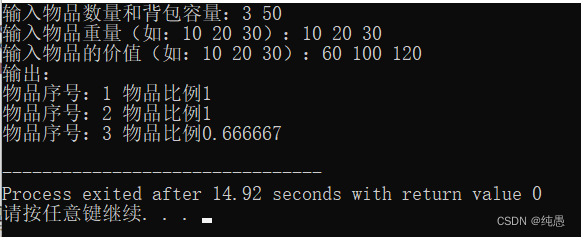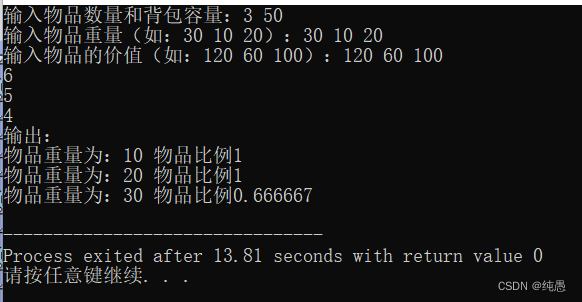请指教！！

展开全文算法
• 贪心算法Q3——01背包问题 /* 问题描述： 有n件物品，每个物品都有一个大小和价值，给你一个固定容量的背包，要求装的东西价值总和最大 实例： 现在有重量分别为35 30 60 50 40 10 25，价值分别为10 40 30 50 35 ...

# 贪心算法Q3——01背包问题

问题描述：
有n件物品，每个物品都有一个大小和价值，给你一个固定容量的背包，要求装的东西价值总和最大
实例：
现在有重量分别为35 30 60 50 40 10 25，价值分别为10 40 30 50 35 40 30的物品，
背包最大承重为150，求该背包所能放置的物品最大价值是多少？

package 贪心;

public class Article {
public int weight,value;
public double valueOfDensity;
Article(int weight,int value){
this.weight=weight;
this.value=value;
this.valueOfDensity=value*1.0/weight;
}
@Override
public String toString() {
return "Article [weight=" + weight + ", value=" + value + ", valueOfDensity=" + valueOfDensity + "]";
}
}


package 贪心;

import java.util.Arrays;
import java.util.Comparator;
import java.util.List;

public class _01背包问题 {
//传一个比较器Comparator<Article> cmp 按设定关键字排序
static void select(String title,Comparator<Article> cmp) {
//将物品变量直接封装在成员方法里作为局部变量 就可以不用通过mian方法传参了
Article [] articles=new Article[] {
new Article(35,10),new Article(30,40),
new Article(60,30),new Article(50,50),
new Article(40,35),new Article(10,40),
new Article(25,30)
};
Arrays.sort(articles, cmp);
//将Article类封装到list存储结构
int capacity=150,weight=0,value=0;
for(int i=0;i<articles.length&&weight<capacity;i++) {
int newWeight=weight+articles[i].weight;
if(newWeight<=capacity) {
weight=newWeight;
value+=articles[i].value;
}
}
for(int i=0;i<selectedArticles.size();i++) {
System.out.println(selectedArticles.get(i));
}
System.out.println(weight);
System.out.println(value);

}
public static void main(String[] args) {
//价值主导
select("价值主导",(Article a1,Article a2)->{
return a2.value-a1.value;
});
//重量主导
select("重量主导",(Article a1,Article a2)->{
return a1.weight-a2.weight;
});
//价值主导
//a2.valueOfDensity-a1.valueOfDensity;结果为double型 而迭代器要求返回类型为int
//Double.compare(double d1,doouble d2)返回int型
select("价值密度主导",(Article a1,Article a2)->{
return Double.compare(a2.valueOfDensity, a1.valueOfDensity);
});

}

}


动态规划版本见：

展开全文• 1、问题描述：给定n种物品和一背包。物品i的重量是wi，其价值为vi，背包的容量为C。问:应如何选择装入背包的物品，使得装入背包中物品的总价值最大?形式化描述：给定c >0, wi >0, vi >0 , 1≤i≤n.要求找一...
• 主要介绍了Python基于贪心算法解决背包问题,简单描述了贪心算法的概念、原理并结合实例形式分析了Python使用贪心算法解决背包问题的具体操作技巧,需要的朋友可以参考下
• 实验三、 0-1 背包问题(贪心算法)实验代码：#includeint max(int a,int b){if(a>b)return a;elsereturn b;}void Knapsack(int *v,int *w,int *x,int c,int n, int m){int i,j;for(j=0;j{if(jm[n][j]=0;...
• 一个贪心算法的比较简单的程序，经运行是可以使用的
• 证明贪心算法的结构 第一步：符合贪心选择的特性（Greedy Choice Property） 我们需要证明我们的第一个选择（贪心选择 Greedy Choice，First Choice）包含在某些最优解中 第二步：符合归纳法结构（Inductive ...数据结构 算法
• 0-1背包问题贪心算法）C语言源程序. 物品名称、物品效益、物品重量、物品的效益重量比等定义了物品的结构体。
• 0-1背包问题贪心算法） 实验代码： #include<stdio.h> int max(int a,int b) { if(a>b) return a; else return b; } void Knapsack(int *v,int *w,int *x,int c,int n, int m) { ...
• 运用贪心策略解决0 1背包问题 void beibao(int *w,int *v,int *x,int n,int *C) { int i,j,temp; for(i=0;i;i++) for(j=i+1;j;j++) if(v[i]/w[i][j]/w[j]) { temp=v[i]; v[i]=v[j]; v[j]=temp...
• 课程的随堂作业，C语言的，用dev就能运行，萌新代码，勿喷，仅仅帮助不想写作业的朋友方便一下，反正老师也不会仔细检查的C语言
• 所谓0/1背包问题是指在物品不能分割，只能整件装入背包或不装入的情况下，求一种最佳装载方案使得总收益最大。 Input 第1行中有2个正整数n（n<=50）和M，表示有n件物品，背包载重为M(m<=100)。然后输入n...动态规划 c语言 c++
• 用3种方法求解0-1背包问题贪心算法、动态规划、分支限界法），获得精确最优解或近似最优解均可。 通过一个规模较大的实例比较不同方法的求解速度，分析不同算法的时间复杂度，并分析是否能获得最优解。 实验结果...动态规划 c++...